# ISEE Middle Level Math : How to find percentage

## Example Questions

### Example Question #61 : Percentage

What is the decimal equivalent of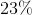?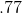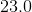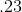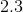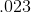Explanation:

To solve, divide the percentage by 100: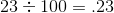Alternatively, you can convert a percentage to a decimal by moving the decimal point two places to the left. Here, 23 can be written as 23.0, and moving the decimal two places to the left gives .23, the same result as above.

Answer:### Example Question #62 : Percentage

What is the decimal equivalent of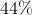?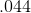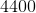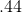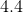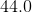Explanation:

To convert to a decimal, divide the percentage by 100, which is really the same as moving the decimal point two places to the left: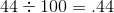Answer:### Example Question #63 : Percentage

What is the decimal equivalent of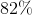?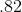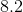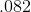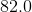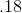Explanation:

To solve, divide the percentage by 100, i.e. move the decimal point two places to the left: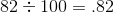Answer:### Example Question #64 : Percentage

What is the decimal equivalent of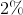?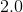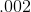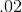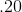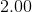Explanation:

To solve, divide the percentage by 100, or move the decimal point two places to the left: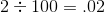Answer:### Example Question #65 : Percentage

What is the decimal equivalent of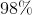?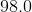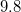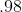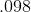Explanation:

To solve, divide the percentage by 100, or move the decimal point two places to the left: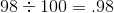Answer:### Example Question #66 : Percentage

Convert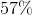to a decimal.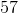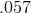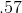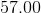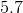Explanation:

Divide the percentage by 100, or move the decimal point two places to the left: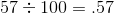Answer:### Example Question #67 : Percentage

What is the decimal equivalent of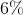?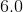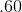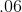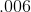Explanation:

To solve, divide the percentage by 100: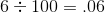This is really just the same as moving the decimal point in 6.0% two places to the left.

Answer:### Example Question #68 : Percentage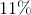is equivalent to ____________.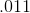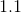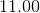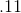Explanation:

To solve, divide the percentage by 100: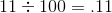Answer:### Example Question #69 : Percentage

What is the decimal equivalent of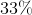?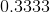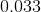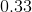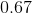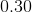Explanation:

To solve, divide by one hundred: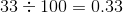Answer:### Example Question #70 : Percentage

What is 35% of 120?To find the percentage of something, you can multiply the decimal equivalent of the percentage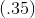by the whole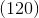. Your answer is.